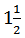Set-24 Arithmetic (Miscellaneous) For SBI PO and SBI Clerk 2019 | Must Go Through These QuestionsDear Aspirants,
We are providing the most important Arithmetic (Miscellaneous) Questions for SBI PO 2019, SBI Clerk 2019 and all other competitive bank and insurance exams. These questions have very high chances to be asked in SBI PO 2019, SBI Clerk 2019.
Get the Best Test Series for SBI PO 2019 at the most affordable price (Based on Real Exam Pattern) – Click Here
Download the Best GK Gaming App for Current Affairs and GK (Bank+SSC)– Click here (App No 1)  (App No 2)

1. Mohan spends 40% of his salary on food items 50% of the remaining on transport, 30% of the remaining, after spending on food and transport, he spends on clothes and saves the balance. If he saves Rs. 630 every month, what is his monthly salary?

2. The average marks of a student in 8 subjects is 87. Of these, the highest marks is 2 more than the one next in value. If these two subjects are eliminated, the average marks of the remaining subjects is 85. What is the highest marks obtained by him?

3. A certain product C is made of two ingredients A and B in the proportion of 2 : 5. The price of A is three times that of B. The overall cost of C is Rs. 5.20 per kg including labour charges of 80 paise per kg. Find the cost of B per kg?

4. A tradesman gives 4% discount on the marked price and 1 article free with every 15 articles bought and still gains 35% The marked price is more than the cost price by:

5. If the selling price of a mat is five times the discount offered and if the percentage of discount is equal to the percentage profit, find the ratio of the discount offered to the cost price.

6. A and B together can do a piece of work in 12 days. B and C together can do the same work in 16 days. After A has been working at it for 5 days and B for 7 days, C finishes it in 13 days. In how many days will C alone be able to do the work?

7. Two men A and B walk from P to Q at a distance of 21 Km at the rates of 3 and 4 km an hour, respectively. B reaches Q and returns immediately and meets A at R. The distance from P to R is:

8. A person has a chemical of Rs. 25 per litre. In what ratio should water be mixed in that chemical, so that after selling the mixture at Rs. 20 per litre he may get a profit of 25%?

9. Pipe A can fill a tank in 3 hrs and 45 minutes. 2 hrs after the pipe started filling the empty tank the motor stopped working. What percent of the tank was left empty?

10. A man swimming in a stream which flowskm/h finds that in a given time he can swim twice as far with the stream as he can against it. At what rate does he swim?

1. Question

Mohan spends 40% of his salary on food items 50% of the remaining on transport, 30% of the remaining, after spending on food and transport, he spends on clothes and saves the balance. If he saves Rs. 630 every month, what is his monthly salary?

Ans: 22. Question

The average marks of a student in 8 subjects is 87. Of these, the highest marks is 2 more than the one next in value. If these two subjects are eliminated, the average marks of the remaining subjects is 85. What is the highest marks obtained by him?

Ans: 13. Question

A certain product C is made of two ingredients A and B in the proportion of 2 : 5. The price of A is three times that of B. The overall cost of C is Rs. 5.20 per kg including labour charges of 80 paise per kg. Find the cost of B per kg?

Ans: 44. Question

A tradesman gives 4% discount on the marked price and 1 article free with every 15 articles bought and still gains 35% The marked price is more than the cost price by:

Ans: 45. Question

If the selling price of a mat is five times the discount offered and if the percentage of discount is equal to the percentage profit, find the ratio of the discount offered to the cost price.

Ans: 46. Question

A and B together can do a piece of work in 12 days. B and C together can do the same work in 16 days. After A has been working at it for 5 days and B for 7 days, C finishes it in 13 days. In how many days will C alone be able to do the work?

Ans: 27. Question

Two men A and B walk from P to Q at a distance of 21 Km at the rates of 3 and 4 km an hour, respectively. B reaches Q and returns immediately and meets A at R. The distance from P to R is:

Ans: 48. Question

A person has a chemical of Rs. 25 per litre. In what ratio should water be mixed in that chemical, so that after selling the mixture at Rs. 20 per litre he may get a profit of 25%?

Ans: 39. Question

Pipe A can fill a tank in 3 hrs and 45 minutes. 2 hrs after the pipe started filling the empty tank the motor stopped working. What percent of the tank was left empty?

Ans: 210. Question

A man swimming in a stream which flowskm/h finds that in a given time he can swim twice as far with the stream as he can against it. At what rate does he swim?

Ans: 1